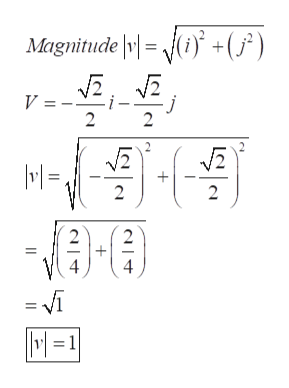# The question says:"Find the magnitude and direction (in degrees) of the vector"V=-((sqrt)2)/2 , -((sqrt)2)/2

Question
5 views

The question says:

"Find the magnitude and direction (in degrees) of the vector"

V=-((sqrt)2)/2 , -((sqrt)2)/2

check_circle

Step 1

Refer to the question , we have to find the magnitude and direction (in degrees) of the vector"
V=-((sqrt)2)/2 , -((sqrt)2)/2.

Step 2

Now to find the magnitude, or length of a...help_outlineImage TranscriptioncloseMagnitude +(/) V = i- 2 2 2 2 2 2 = i M=1 fullscreen

### Want to see the full answer?

See Solution

#### Want to see this answer and more?

Solutions are written by subject experts who are available 24/7. Questions are typically answered within 1 hour.*

See Solution
*Response times may vary by subject and question.
Tagged in

### Other